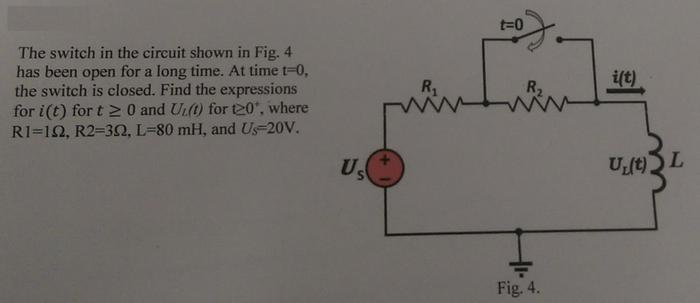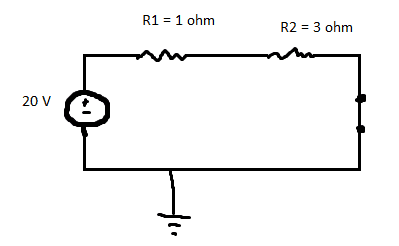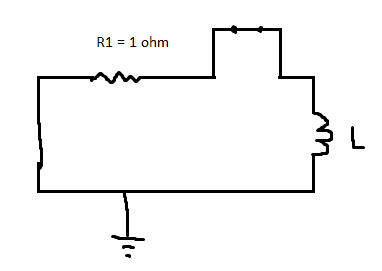# RL circuit problem

• Engineering

## Homework Statement## The Attempt at a Solution

When the t < 0, the switch is open, the inductor acts like a short therefore the circuit looks likeThe current is then 20V / 4 ohms = 5 A
Since the inductor has been in series with the battery for a long time, it is safe to assume it is fully charged? So the current across the inductor is 5 A?

Assuming this is correct, io= 5 A

If we close the switch, R2 becomes shorted, then the current in the circuit would then be 20V / 1 ohm = 20 A

So the change is in current is 20A -5A = 15A
I don't know if we need this value, but inductors resist change in current so it probably is important?____________________________

when t = 0, switch is closed and battery becomes shorted and R2 becomes shorted.Current in circuit is now 20A.
To find voltage change in inductor, we will probably need the change in current and the time for current change.

I'm guessing to find the time, we will need I(t) = Io e^-t/(L/R))
time constant when switch is closed is L/R = 0.08H / 1 ohm = 0.08 sec

20 A = 5A (e^-t/(0.08))

4 = e-12.5t = e1/12.5t

ln 4 = 1/12.5t

1.386* 12.5= 17.32 = 1/t

t= 0.0577 sec

Am I on the right path so far?

cnh1995
Homework Helper
Gold Member
I'm guessing to find the time, we will need I(t) = Io e^-t/(L/R))
time constant when switch is closed is L/R = 0.08H / 1 ohm = 0.08 sec

20 A = 5A (e^-t/(0.08))

4 = e-12.5t = e1/12.5t

ln 4 = 1/12.5t

1.386* 12.5= 17.32 = 1/t

t= 0.0577 sec

Am I on the right path so far?
You are asked to find i(t). Initially, the current is 5A. Long after the switch is closed, the current is 20A. You need to write an exponential equation which gives i=5A at t=0 and i=20A at t=∞.

You are asked to find i(t). Initially, the current is 5A. Long after the switch is closed, the current is 20A. You need to write an exponential equation which gives i=5A at t=0 and i=20A at t=∞.
ok, I think I found it.

i(t) = i(∞) - Δi(e^-t/(L/R) = 20 - 15(e^-t/(L/R))

cnh1995
Homework Helper
Gold Member
ok, I think I found it.

i(t) = i(∞) - Δi(e^-t/(L/R) = 20 - 15(e^-t/(L/R))
Right. It can be derived using differential equation.

Right. It can be derived using differential equation.
I didn't take differential equations so I'm not too good with that.

cnh1995
Homework Helper
Gold Member
I didn't take differential equations so I'm not too good with that.
That won't present much problem as far as you can remember these exponential equations correctly.

now solving for UL(t) = L di/dt

= L *[ i(∞) - Δi(e^-t/(L/R) = L* [ 20 - 15(e^-t/(L/R))) ]

Time constant of when switch is closed is L/R
Req is just the 1 ohm resistor

0.08H / 1 ohm = 0.08 sec

= L *[ i(∞) - Δi(e^-t/(L/R)

= 0.08 [20 - 15(e^-12.5t) ]

cnh1995
Homework Helper
Gold Member
now solving for UL(t) = L di/dt

L = L *[ i(∞) - Δi(e^-t/(L/R) = 20 - 15(e^-t/(L/R))) ]
You'll need to calculate di/dt for that.
There is another way, without involving differentiation.

You'll need to calculate di/dt for that.
There is another way, without involving differentiation.
so when t < 0, current through inductor is 5, but change in current is 0, so no voltage drop.

when t = 0 , current through inductor increases from 5 to 20, so there will be a voltage drop.

when t →∞, current is steady again, therefore voltage drop across inductor is 0 again.

when the switch is closed, the voltage source becomes shorted and the inductor is in series with R1.

V = I R = 5A 1ohm = 5 volts at t = 0

Vo = 5 volts

thus V(t)= Vo (e^-t/0.08)

is this correct?

cnh1995
Homework Helper
Gold Member
when t = 0 , current through inductor increases from 5 to 20,
Current doesn't increase from 5A to 20A instantly. At t=0, current through the inductor is still 5A and it rises exponentially according to the equation you used earlier. Remember, inductor opposes sudden change in current.
when the switch is closed, the voltage source becomes shorted
No, R2 gets shorted.
To maintain the current at 5A at t=0, back emf of 15V is induced in the inductor.
So, VL=15V at t=0.
You have i(t) and you know R1, Us. You can get the expression for UL using
the equation UL+UR1=Us.

Current doesn't increase from 5A to 20A instantly. At t=0, current through the inductor is still 5A and it rises exponentially according to the equation you used earlier. Remember, inductor opposes sudden change in current.

No, R2 gets shorted.
To maintain the current at 5A at t=0, back emf of 15V is induced in the inductor.
So, VL=15V at t=0.
You have i(t) and you know R1, Us. You can get the expression for UL using
the equation UL+UR1=Us.
i thought R2 and the voltage source were shorted.

why isn't voltage source shorted? I thought it had to be shorted to find Req

cnh1995
Homework Helper
Gold Member
why isn't voltage source shorted? I thought it had to be shorted to find Req
You need not short the voltage source as it is clear that equivalent resistance is R1, since all the current now passes through R1. For calculation of Thevenin equivent from inductor terminals, yes you should short the voltage source but while writing expression for current or voltage, you should include it back.

•goonking
i(t) = 20 +(5-20) e-12.5t

the voltage drop across R1 is still (5A)(1ohm) = 5 volts (because current in circuit is still 5A, but will increase to 20A over time) at t=0, but will exponentially increase to 20 volts over time.

Since the voltage drop on R1 is 5 volts, and the battery supplies 20 volts, the inductor must have the remaining 15 volts (at t=0) which will decrease exponentially to 0 volts over time.

so for UL(t)= UL(o) e-12.5t = 15e-12.5t

cnh1995
Homework Helper
Gold Member
i(t) = 20 +(5-20) e-12.5t

the voltage drop across R1 is still (5A)(1ohm) = 5 volts (because current in circuit is still 5A, but will increase to 20A over time) at t=0, but will exponentially increase to 20 volts over time.

Since the voltage drop on R1 is 5 volts, and the battery supplies 20 volts, the inductor must have the remaining 15 volts (at t=0) which will decrease exponentially to 0 volts over time.

so for UL(t)= UL(o) e-12.5t = 15e-12.5t
Looks good!

•goonking